# 循环神经网络（RNN）原理通俗解释

## 1.RNN怎么来的？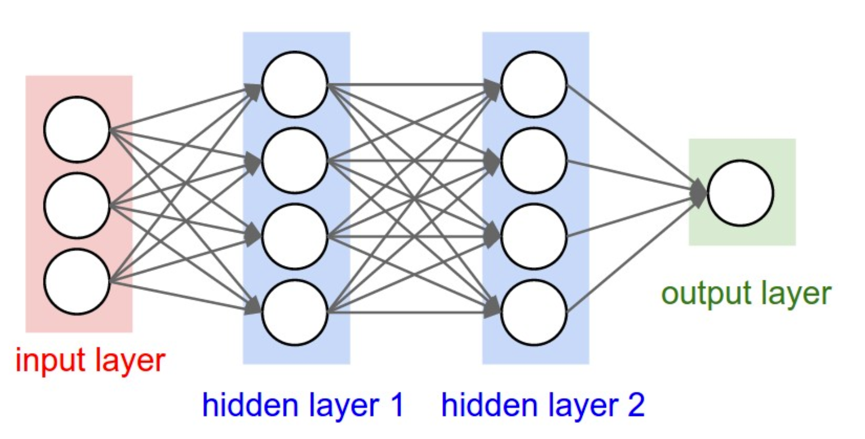## 2.RNN的网络结构及原理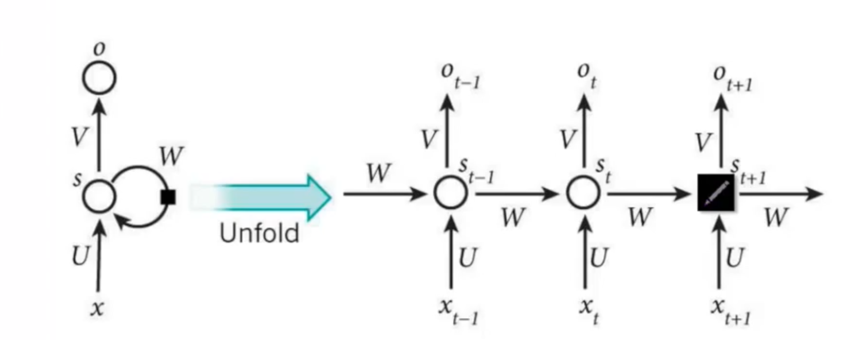RNN是一个序列到序列的模型，假设 xt1,xt,xt+1 x t − 1 , x t , x t + 1 $x_{t-1},x_{t},x_{t+1}$是一个输入：“我是中国“，那么 ot1,ot o t − 1 , o t $o_{t-1},o_{t}$就应该对应”是”，”中国”这两个，预测下一个词最有可能是什么？就是 ot+1 o t + 1 $o_{t+1}$应该是”人”的概率比较大。

Xt:tot:tSt:t X t : 表 示 t 时 刻 的 输 入 ， o t : 表 示 t 时 刻 的 输 出 ， S t : 表 示 t 时 刻 的 记 忆
。因为我们当前时刻的输出是由记忆和当前时刻的输入决定的，就像你现在大四，你的知识是由大四学到的知识（当前输入）和大三以及大三以前学到的东西的（记忆）的结合，RNN在这点上也类似，神经网络最擅长做的就是通过一系列参数把很多内容整合到一起，然后学习这个参数，因此就定义了RNN的基础：
St=f(UXt+WSt1) S t = f ( U ∗ X t + W ∗ S t − 1 )

ot=softmax(VSt) o t = s o f t m a x ( V S t )

RNN中的结构细节：
1.可以把 St S t $S_t$当作隐状态，捕捉了之前时间点上的信息。就像你去考研一样，考的时候记住了你能记住的所有信息。
2. ot o t $o_t$是由当前时间以及之前所有的记忆得到的。就是你考研之后做的考试卷子，是用你的记忆得到的。
3.很可惜的是， St S t $S_t$并不能捕捉之前所有时间点的信息。就像你考研不能记住所有的英语单词一样。
4.和卷积神经网络一样，这里的网络中每个cell都共享了一组参数（U，V，W）,这样就能极大的降低计算量了。
5. ot o t $o_t$在很多情况下都是不存在的，因为很多任务，比如文本情感分析，都是只关注最后的结果的。就像考研之后选择学校，学校不会管你到底怎么努力，怎么心酸的准备考研，而只关注你最后考了多少分。

## 3.RNN的改进1：双向RNN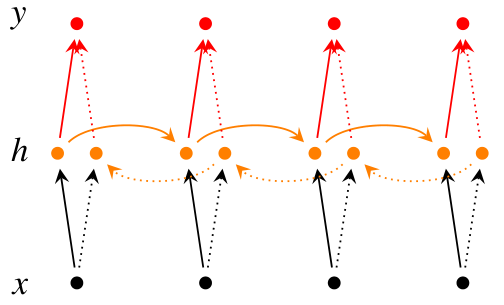S1t=f(U1Xt+W1St1+b1) 从 前 往 后 ： S t 1 → = f ( U 1 → ∗ X t + W 1 → ∗ S t − 1 + b 1 → )
:S2t=f(U2Xt+W2St1+b2) 从 后 往 前 : S t 2 → = f ( U 2 ∗ X t → + W 2 → ∗ S t − 1 + b 2 → )
ot=softmax(V[S1t;S2t]) 输 出 ： o t = s o f t m a x ( V ∗ [ S t 1 → ; S t 2 → ] )

## 4.RNN的改进2：深层双向RNN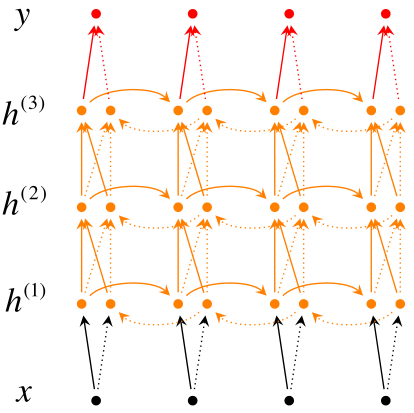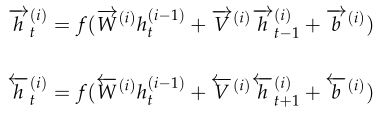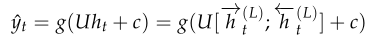### 4.1 Pyramidal RNN## 5.RNN的训练-BPTT

${s}_{t}=tanh\left(U{x}_{t}+W{s}_{t-1}\right)$

ŷ t=softmax(Vst) y ^ t = s o f t m a x ( V s t )

tEt(yt,ŷ t)=ytlogŷ t t 时 刻 的 损 失 ： E t ( y t , y ^ t ) = − y t l o g y ^ t

E(yt,ŷ t)=tEt(yt,ŷ t)=tytlogŷ t E ( y t , y ^ t ) = ∑ t E t ( y t , y ^ t ) = − ∑ t y t l o g y ^ t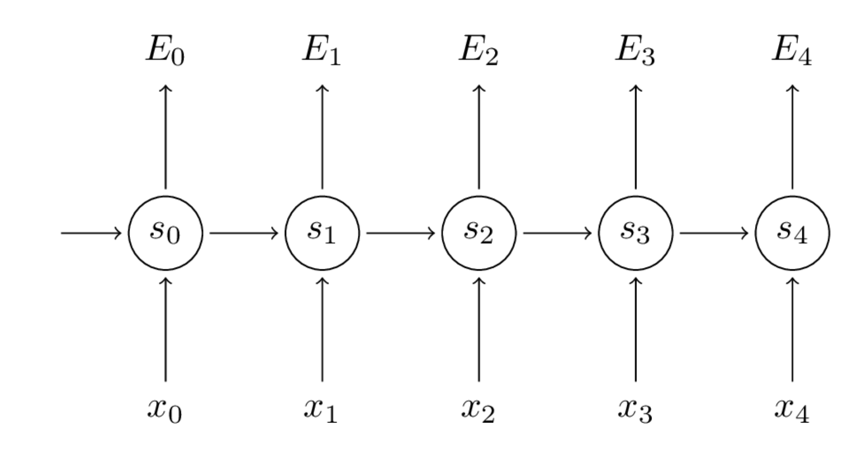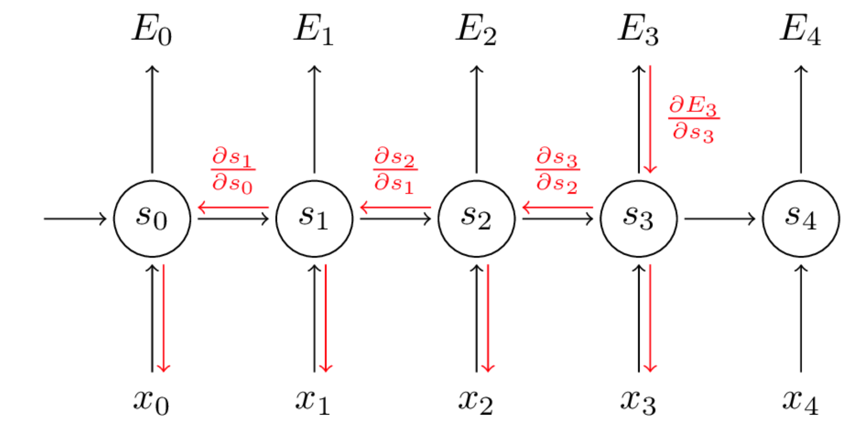E3W=E3ŷ 3ŷ 3s3s3W ∂ E 3 ∂ W = ∂ E 3 ∂ y ^ 3 ∂ y ^ 3 ∂ s 3 ∂ s 3 ∂ W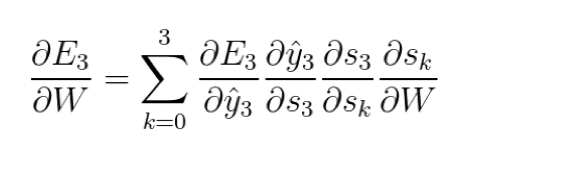## 6.RNN与CNN的结合应用：看图说话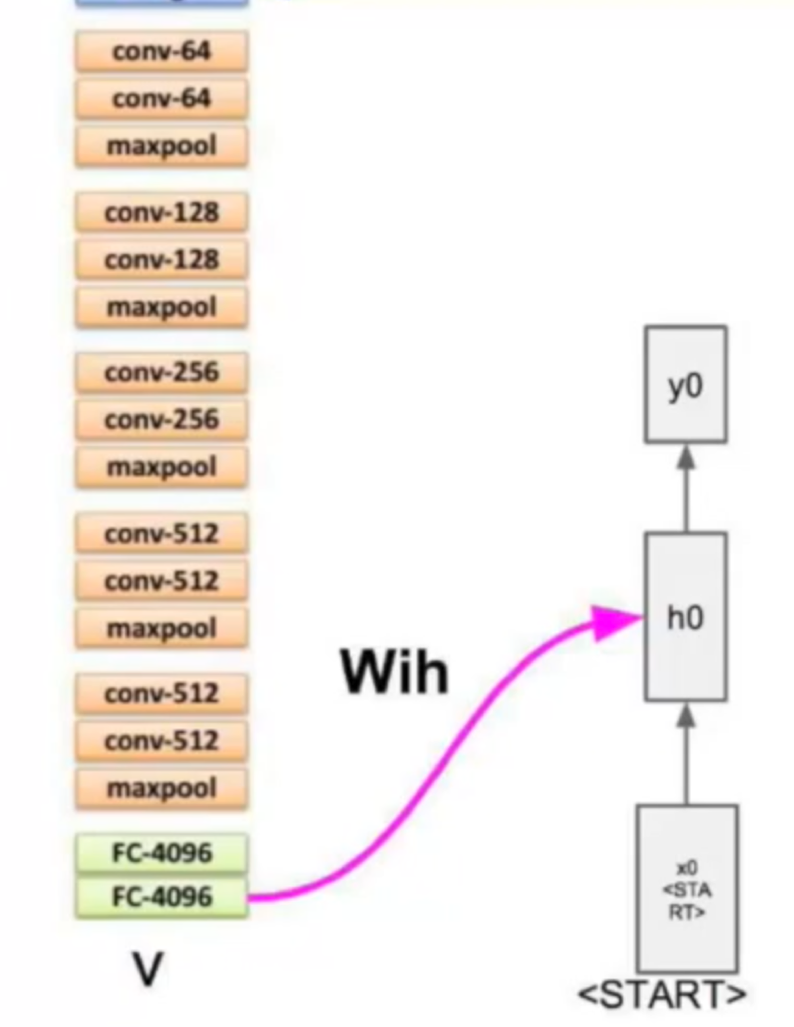St=tanh(UXt+WSt1) S t = t a n h ( U ∗ X t + W ∗ S t − 1 )

St=tanh(UXt+WSt1+VX) S t = t a n h ( U ∗ X t + W ∗ S t − 1 + V ∗ X )

## 7.RNN项目练手

RNN可以写歌词，写诗等，这有个项目可以玩玩，还不错。
Tensorflow实现RNN

02-1006-112万+
09-2345万+
06-156390
06-0625万+
05-2811万+
09-012万+
07-271万+
03-204177
10-121382
01-181万+
07-126010
01-091883
07-2113万+
10-09710
©️2020 CSDN 皮肤主题: 书香水墨 设计师:CSDN官方博客